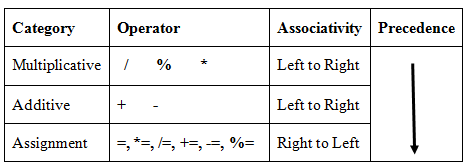## Precedence and Associativity of operators (Arithmetic and Assignment operators)

View

Precedence and Associativity of Arithmetic and Assignment operators are shown in Table 1 below-Table 1

Example

int a = 20;
int b = 10;
int c = 15;
int d = 5;
int e;
e =  (a + b) * c / d;
=  (20+10) * 15/5
=  30 * 15/5
=  450 /5
=  90

e =  a + (b * c) / d;
=  20+ (10 * 15)/5
=  20 + 150/5
=  20 + 30
e =  50